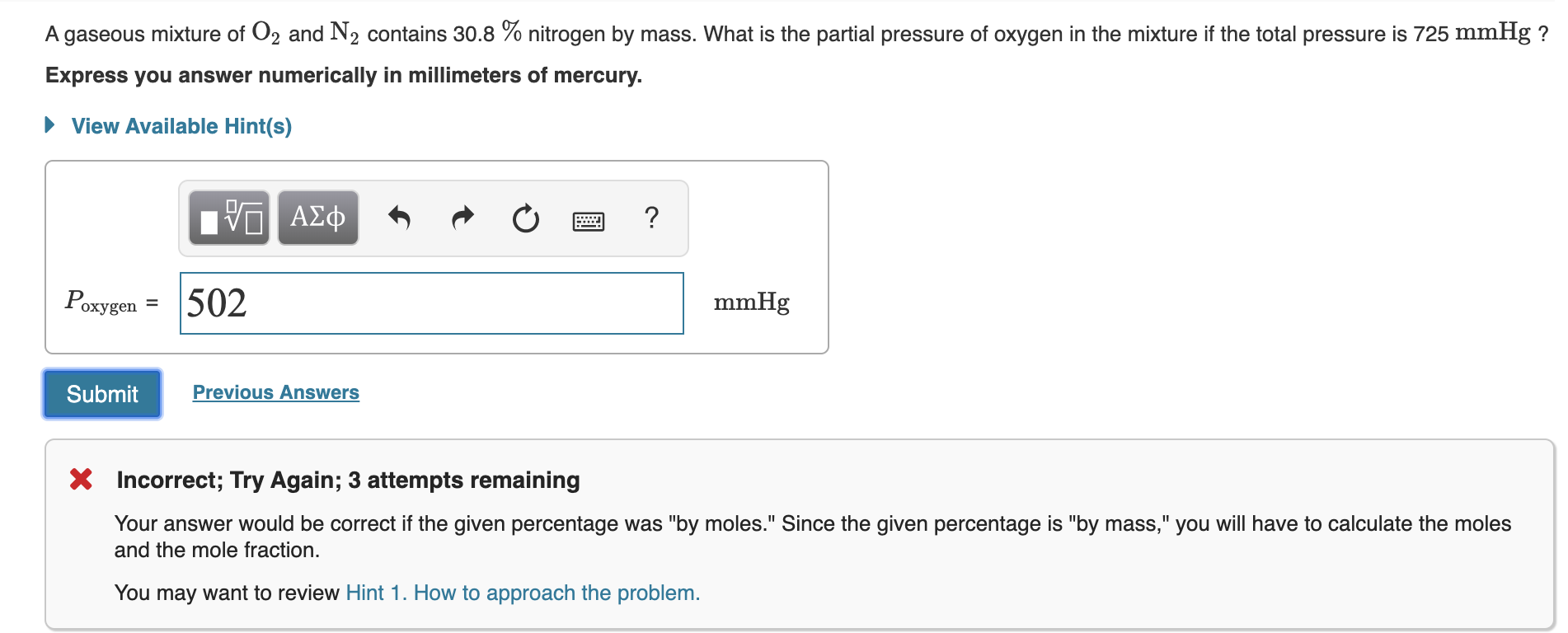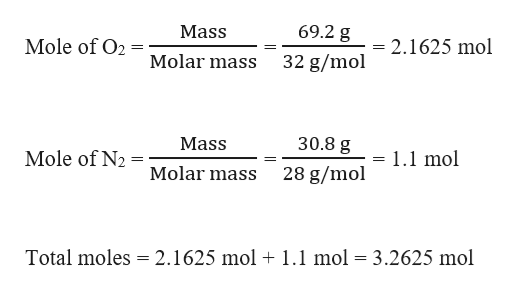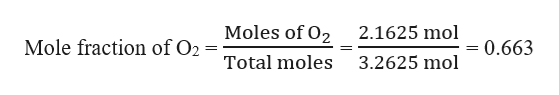# A gaseous mixture of O2 and N2 contains 30.8 % nitrogen by mass. What is the partial pressure of oxygen in the mixture if the total pressure is 725 mmHg ?Express you answer numerically in millimeters of mercury.View Available Hint(s)ΑΣφ502PoxygenmmHgохуgPrevious AnswersSubmitIncorrect; Try Again; 3 attempts remainingYour answer would be correct if the given percentage was "by moles." Since the given percentage is "by mass," you will have to calculate the molesand the mole fraction.You may want to review Hint 1. How to approach the problem.

Question
329 viewshelp_outlineImage TranscriptioncloseA gaseous mixture of O2 and N2 contains 30.8 % nitrogen by mass. What is the partial pressure of oxygen in the mixture if the total pressure is 725 mmHg ? Express you answer numerically in millimeters of mercury. View Available Hint(s) ΑΣφ 502 Poxygen mmHg охуg Previous Answers Submit Incorrect; Try Again; 3 attempts remaining Your answer would be correct if the given percentage was "by moles." Since the given percentage is "by mass," you will have to calculate the moles and the mole fraction. You may want to review Hint 1. How to approach the problem. fullscreen
check_circle

star
star
star
star
star
1 Rating
Step 1

Given,

Total pressure of the mixture = 725 mmHg

Gaseous mixture of O2 and N2 contains mass % of N2 = 30.8 %

This means that in total 100 g of the mixture, there is 30.8 g of N2.

⸫ Mass of O2 = 100 g – 30.8 g = 69.2 g

Step 2

Moles of O2 and N2 can be calculated as:help_outlineImage Transcriptionclose69.2 g Mass Mole of O2 2.1625 mol Molar mass 32 g/mol 30.8 g Mass Mole of N2 1.1 mol Molar mass 28 g/mol Total moles 2.1625 mol + 1.1 mol = 3.2625 mol fullscreen
Step 3

Mole fraction of O2 can be c...help_outlineImage TranscriptioncloseMoles of O2 2.1625 mol Mole fraction of O2 0.663 3.2625 mol Total moles fullscreen

### Want to see the full answer?

See Solution

#### Want to see this answer and more?

Solutions are written by subject experts who are available 24/7. Questions are typically answered within 1 hour.*

See Solution
*Response times may vary by subject and question.
Tagged in

### General Chemistry